# RD Sharma Solutions for Class 10 Maths Chapter 15 Areas Related To Circles Exercise 15.2

This exercise primarily deals with problems about the sector of a circle and its area. The RD Sharma Solutions Class 10 can be of great use for students to learn the correct procedure to solving any problem from the book. Further, students can also download the RD Sharma Solutions for Class 10 Maths Chapter 15 Areas Related To Circles Exercise 15.2 PDF provided below.

## RD Sharma Solutions for Class 10 Maths Chapter 15 Areas Related To Circles Exercise 15.2 Download PDF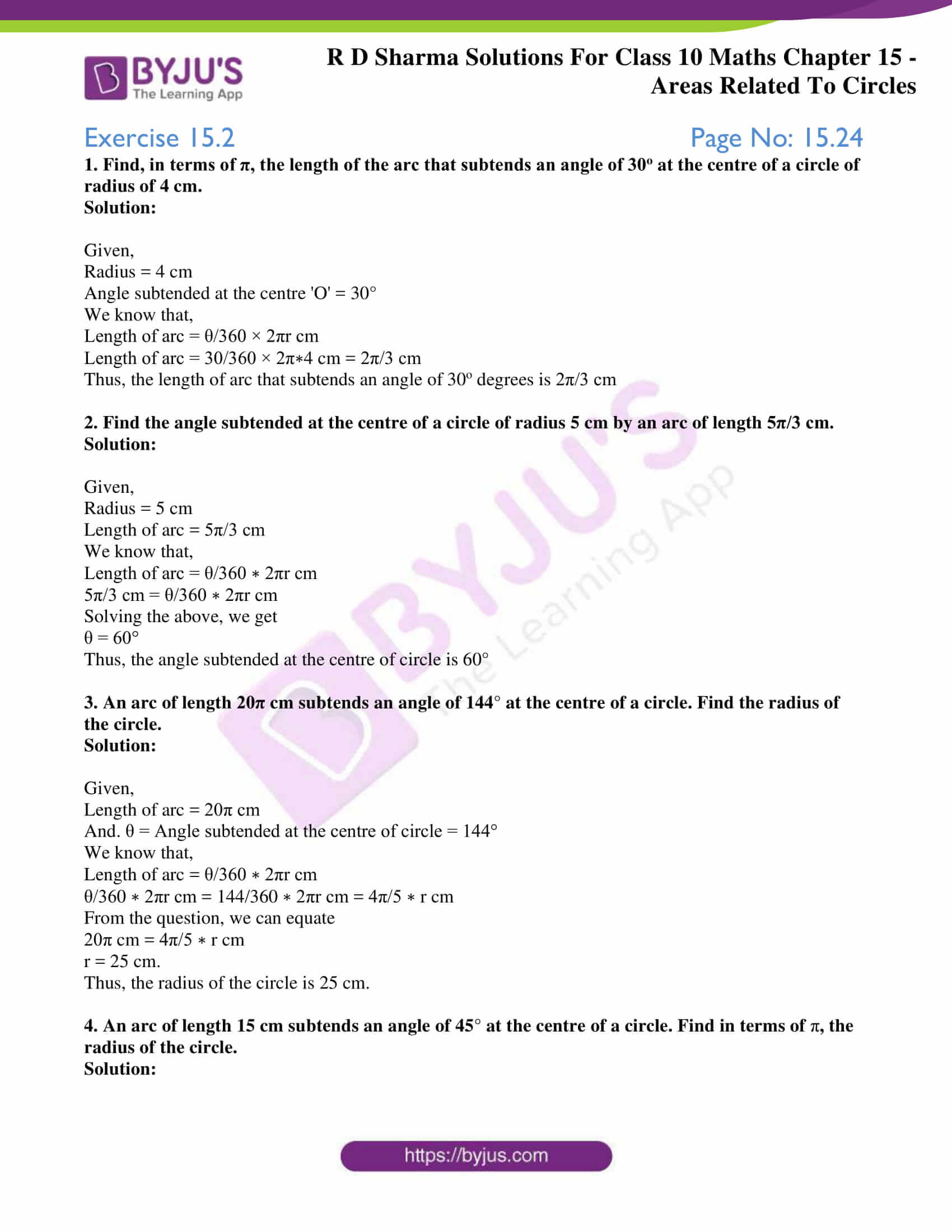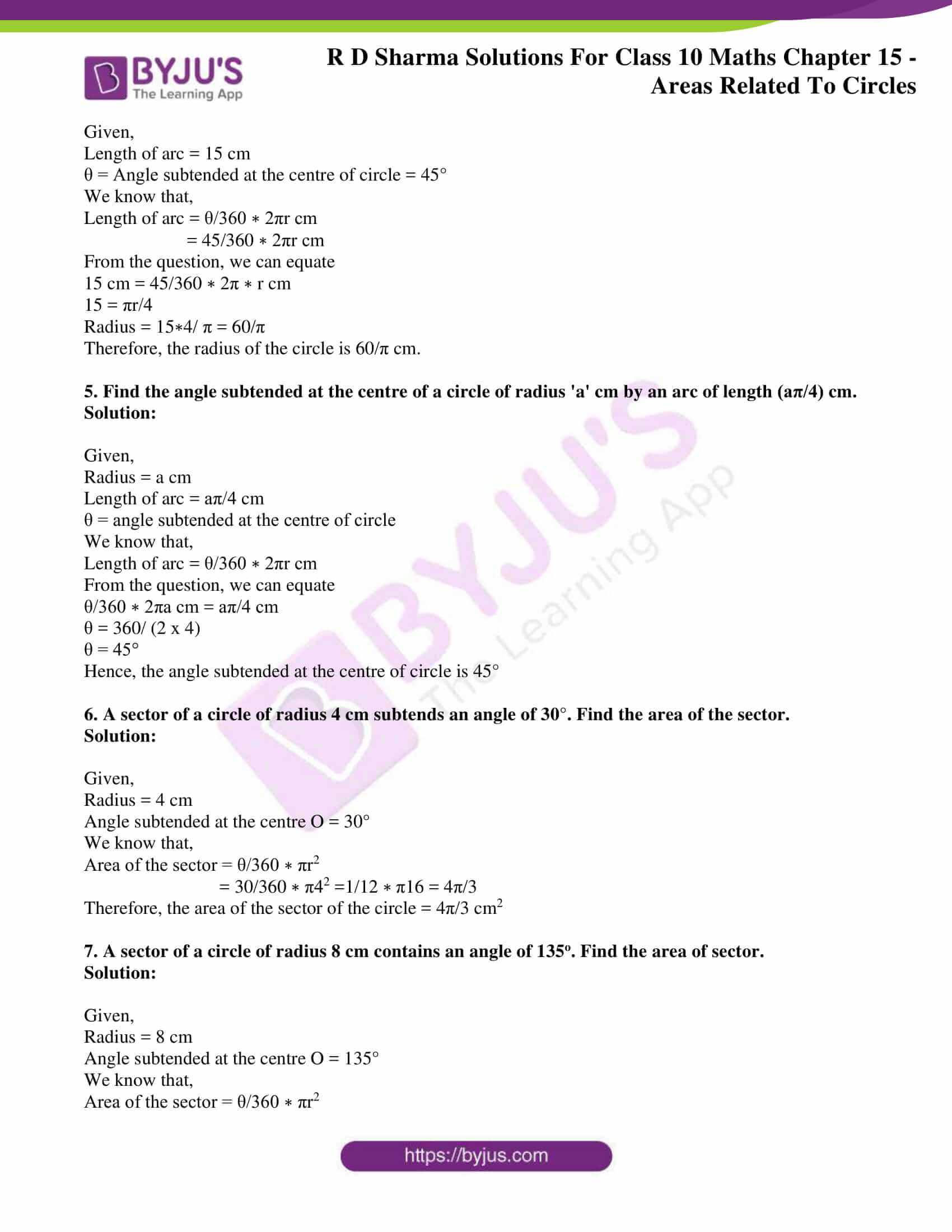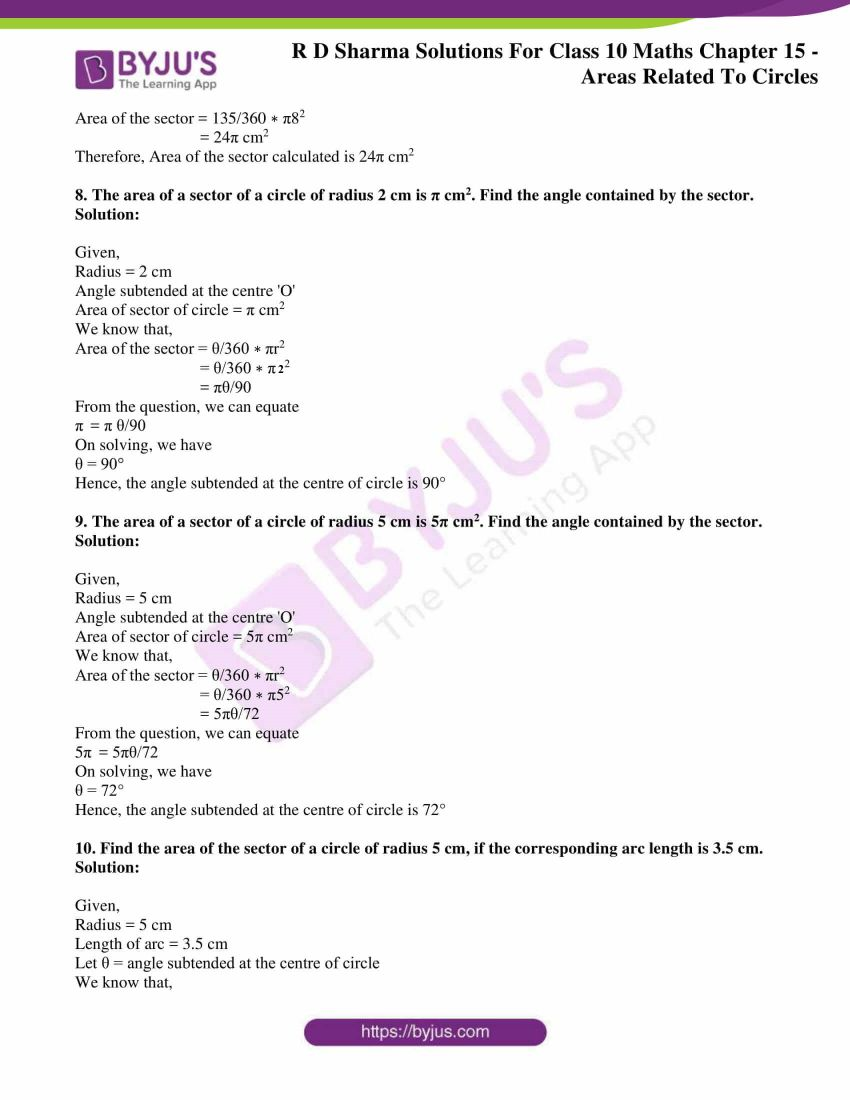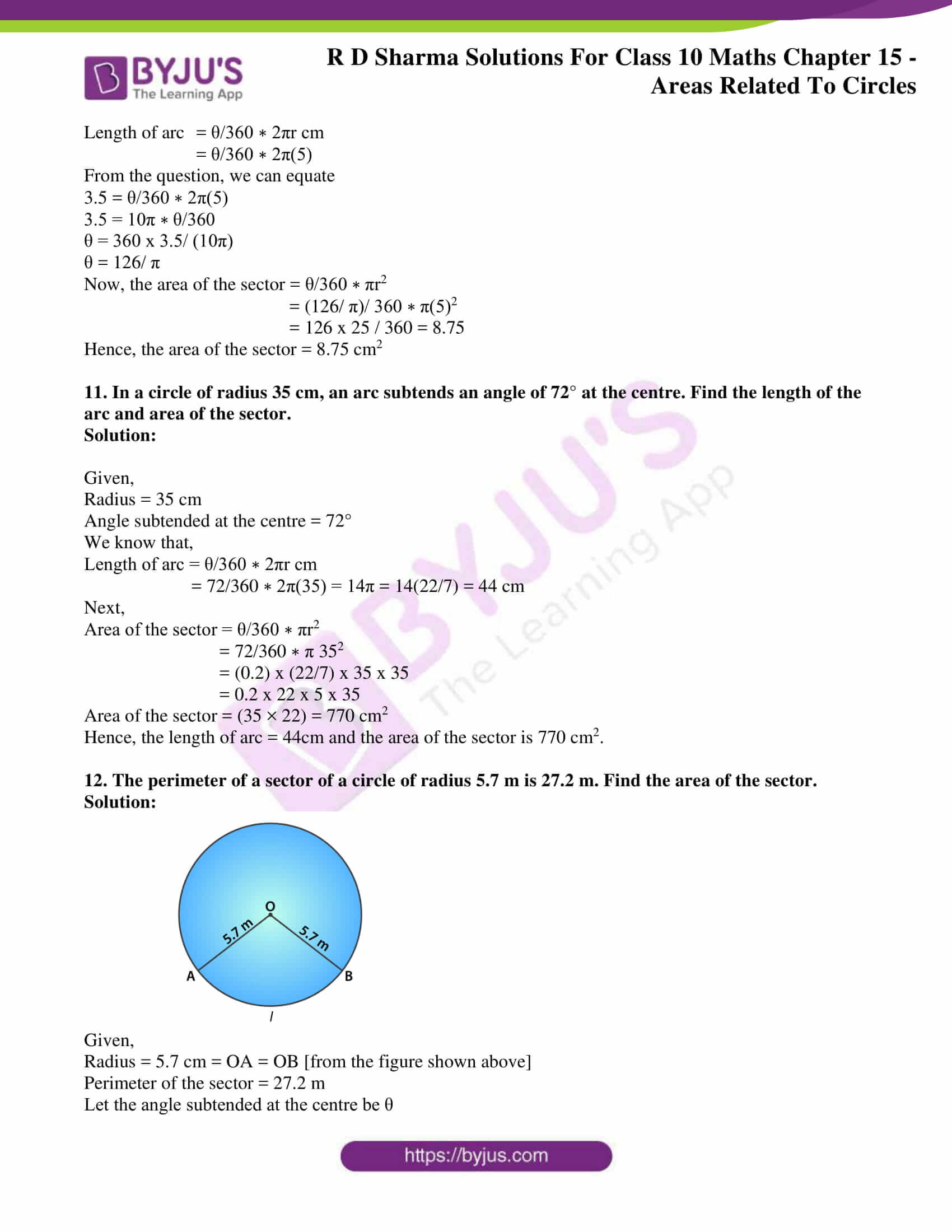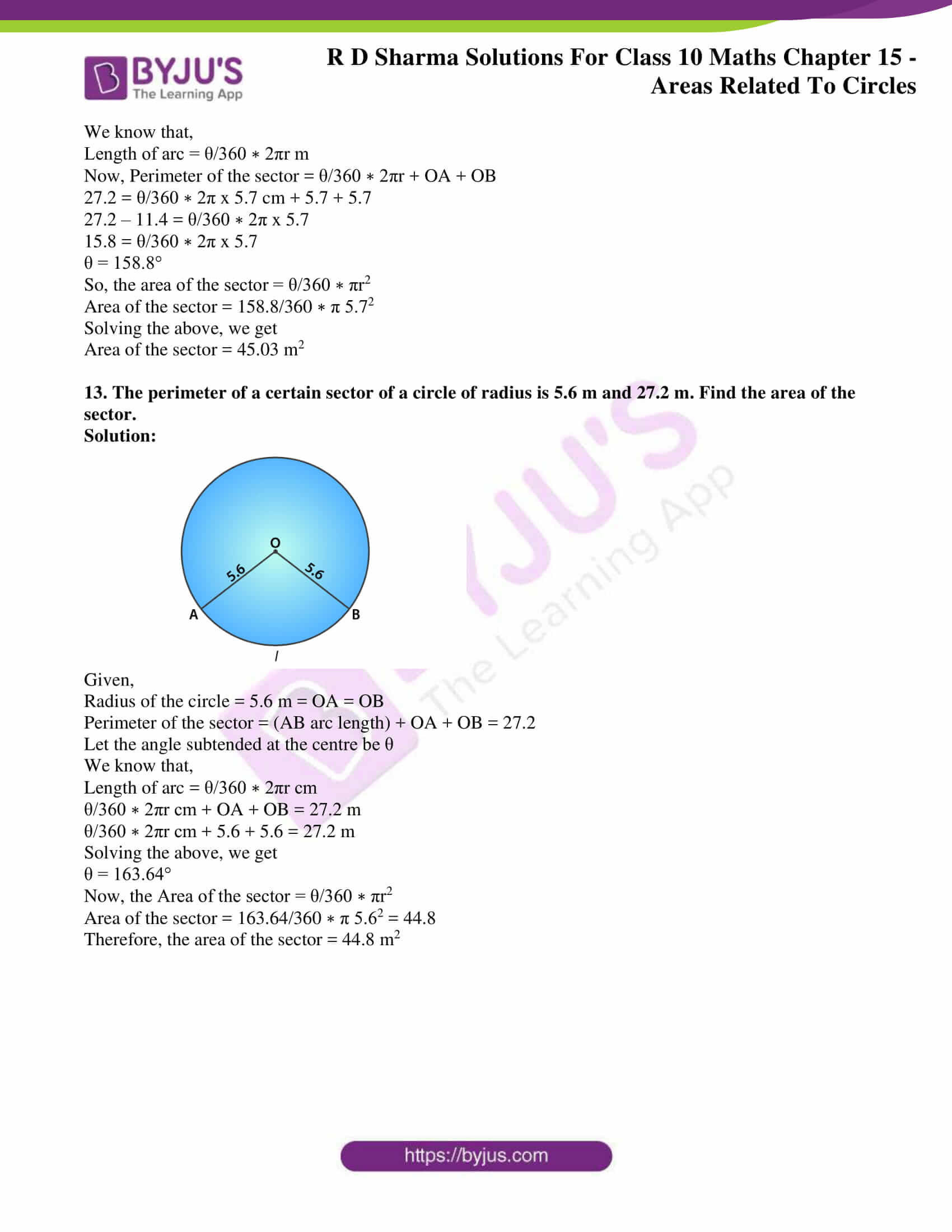### Access RD Sharma Solutions for Class 10 Maths Chapter 15 Areas Related To Circles Exercise 15.2

1. Find, in terms of π, the length of the arc that subtends an angle of 30o at the centre of a circle of radius of 4 cm.

Solution:

Given,

Angle subtended at the centre ‘O’ = 30°

We know that,

Length of arc = θ/360 × 2πr cm

Length of arc = 30/360 × 2π∗4 cm = 2π/3 cm

Thus, the length of arc that subtends an angle of 30o degrees is 2π/3 cm

2. Find the angle subtended at the centre of a circle of radius 5 cm by an arc of length 5π/3 cm.

Solution:

Given,

Length of arc = 5π/3 cm

We know that,

Length of arc = θ/360 ∗ 2πr cm

5π/3 cm = θ/360 ∗ 2πr cm

Solving the above, we get

θ = 60°

Thus, the angle subtended at the centre of circle is 60°

3. An arc of length 20π cm subtends an angle of 144° at the centre of a circle. Find the radius of the circle.

Solution:

Given,

Length of arc = 20π cm

And. θ = Angle subtended at the centre of circle = 144°

We know that,

Length of arc = θ/360 ∗ 2πr cm

θ/360 ∗ 2πr cm = 144/360 ∗ 2πr cm = 4π/5 ∗ r cm

From the question, we can equate

20π cm = 4π/5 ∗ r cm

r = 25 cm.

Thus, the radius of the circle is 25 cm.

4. An arc of length 15 cm subtends an angle of 45° at the centre of a circle. Find in terms of π, the radius of the circle.

Solution:

Given,

Length of arc = 15 cm

θ = Angle subtended at the centre of circle = 45°

We know that,

Length of arc = θ/360 ∗ 2πr cm

= 45/360 ∗ 2πr cm

From the question, we can equate

15 cm = 45/360 ∗ 2π ∗ r cm

15 = πr/4

Radius = 15∗4/ π = 60/π

Therefore, the radius of the circle is 60/π cm.

5. Find the angle subtended at the centre of a circle of radius ‘a’ cm by an arc of length (aπ/4) cm.

Solution:

Given,

Length of arc = aπ/4 cm

θ = angle subtended at the centre of circle

We know that,

Length of arc = θ/360 ∗ 2πr cm

From the question, we can equate

θ/360 ∗ 2πa cm = aπ/4 cm

θ = 360/ (2 x 4)

θ = 45°

Hence, the angle subtended at the centre of circle is 45°

6. A sector of a circle of radius 4 cm subtends an angle of 30°. Find the area of the sector.

Solution:

Given,

Angle subtended at the centre O = 30°

We know that,

Area of the sector = θ/360 ∗ πr2

= 30/360 ∗ π42 =1/12 ∗ π16 = 4π/3

Therefore, the area of the sector of the circle = 4π/3 cm

7. A sector of a circle of radius 8 cm contains an angle of 135o. Find the area of sector.

Solution:

Given,

Angle subtended at the centre O = 135°

We know that,

Area of the sector = θ/360 ∗ πr2

Area of the sector = 135/360 ∗ π82

= 24π cm2

Therefore, Area of the sector calculated is 24π cm2

8. The area of a sector of a circle of radius 2 cm is π cm2. Find the angle contained by the sector.

Solution:

Given,

Angle subtended at the centre ‘O’

Area of sector of circle = π cm2

We know that,

Area of the sector = θ/360 ∗ πr2

= θ/360 ∗ π22

= πθ/90

From the question, we can equate

π  = π θ/90

On solving, we have

θ = 90°

Hence, the angle subtended at the centre of circle is 90°

9. The area of a sector of a circle of radius 5 cm is 5π cm2. Find the angle contained by the sector.

Solution:

Given,

Angle subtended at the centre ‘O’

Area of sector of circle = 5π cm2

We know that,

Area of the sector = θ/360 ∗ πr2

= θ/360 ∗ π52

= 5πθ/72

From the question, we can equate

= 5πθ/72

On solving, we have

θ = 72°

Hence, the angle subtended at the centre of circle is 72°

10. Find the area of the sector of a circle of radius 5 cm, if the corresponding arc length is 3.5 cm.

Solution:

Given,

Length of arc = 3.5 cm

Let θ = angle subtended at the centre of circle

We know that,

Length of arc = θ/360 ∗ 2πr cm

= θ/360 ∗ 2π(5)

From the question, we can equate

3.5 = θ/360 ∗ 2π(5)

3.5 = 10π ∗ θ/360

θ = 360 x 3.5/ (10π)

θ = 126/ π

Now, the area of the sector = θ/360 ∗ πr2

= (126/ π)/ 360 ∗ π(5)2

= 126 x 25 / 360 = 8.75

Hence, the area of the sector = 8.75 cm2

11. In a circle of radius 35 cm, an arc subtends an angle of 72° at the centre. Find the length of the arc and area of the sector.

Solution:

Given,

Angle subtended at the centre = 72°

We know that,

Length of arc = θ/360 ∗ 2πr cm

= 72/360 ∗ 2π(35) = 14π = 14(22/7) = 44 cm

Next,

Area of the sector = θ/360 ∗ πr2

= 72/360 ∗ π 352

= (0.2) x (22/7) x 35 x 35

= 0.2 x 22 x 5 x 35

Area of the sector = (35 × 22) = 770 cm2

Hence, the length of arc = 44cm and the area of the sector is 770 cm2.

12. The perimeter of a sector of a circle of radius 5.7 m is 27.2 m. Find the area of the sector.

Solution: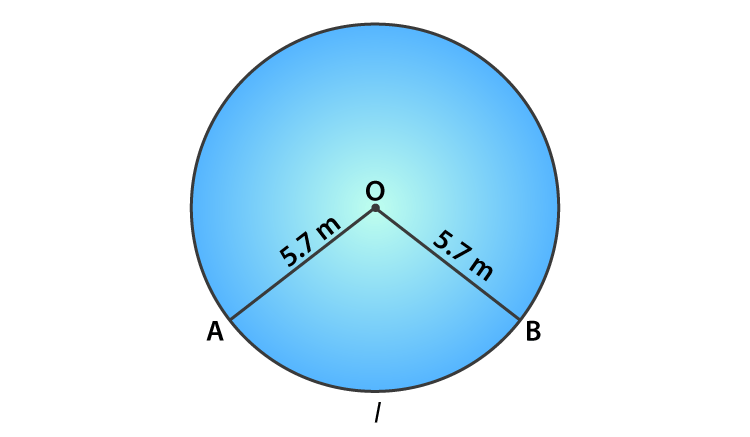Given,

Radius = 5.7 cm = OA = OB [from the figure shown above]

Perimeter of the sector = 27.2 m

Let the angle subtended at the centre be θ

We know that,

Length of arc = θ/360 ∗ 2πr m

Now, Perimeter of the sector = θ/360 ∗ 2πr + OA + OB

27.2 = θ/360 ∗ 2π x 5.7 cm + 5.7 + 5.7

27.2 – 11.4 = θ/360 ∗ 2π x 5.7

15.8 = θ/360 ∗ 2π x 5.7

θ = 158.8°

So, the area of the sector = θ/360 ∗ πr2

Area of the sector = 158.8/360 ∗ π 5.72

Solving the above, we get

Area of the sector = 45.03 m2

13. The perimeter of a certain sector of a circle of radius is 5.6 m and 27.2 m. Find the area of the sector.

Solution: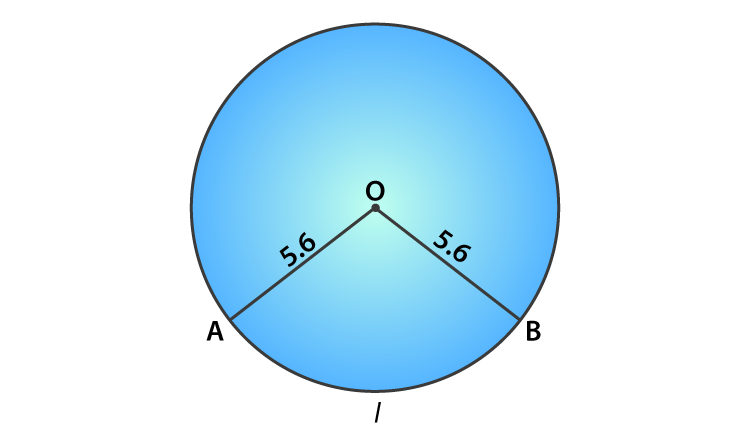Given,

Radius of the circle = 5.6 m = OA = OB

Perimeter of the sector = (AB arc length) + OA + OB = 27.2

Let the angle subtended at the centre be θ

We know that,

Length of arc = θ/360 ∗ 2πr cm

θ/360 ∗ 2πr cm + OA + OB = 27.2 m

θ/360 ∗ 2πr cm + 5.6 + 5.6 = 27.2 m

Solving the above, we get

θ = 163.64°

Now, the Area of the sector = θ/360 ∗ πr2

Area of the sector = 163.64/360 ∗ π 5.62 = 44.8

Therefore, the area of the sector = 44.8 m2